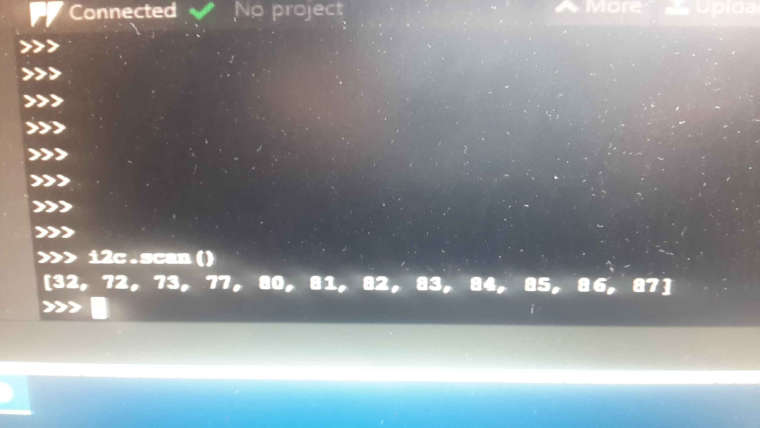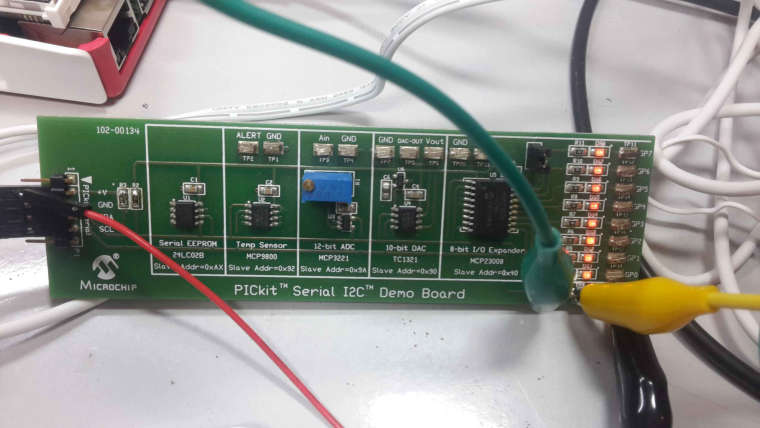# question about I2C address BUS.

• Hello mates!:

I am learning to use the I2C bus, as I understand, the addresses of the devices are HEX addresses.

I'm doing the tests with a Lopy, and a test of several I2C devices.

the test plate marks devices at the addresses 0x40 (mcp23008), 0x90 (tc1321), 0x9A (mcp3221), 0x92 (mcp9800) and 0xAx (24lc02B).

the case that when doing the command.
i2c.scan ()

the addresses do not return them to me in HEX, but neither in its decimal equivalent (which is how I understand that it must return).

after investigating, it turns out that the addresses that return me are in decimal divided by 2.

in this case and knowing it I can handle it, but I would love to understand ...

P.S:

out of this topic, on the same bus I have a raspberry and when I scan it does not give me values that match me!

2_1543500658260_03.jpg• very, very thanks mates!!

now i understand fine!!

• Don't worry, there is always some confusion regarding the i2c addresses.

Chip designers tend to think about i2c devices in terms of two addresses: one to write (xxxxxxx1) and one to read (xxxxxxx0). This is most simple and easy to code.

But the designers at Philips, who invented the I²C bus, thought in a different way. They defined only the first seven bits as "the address". This was, however, happily ignored by the programming community for many years.

With the invention of i2c-OBJECTs, the nearly dead Philips convention was suddenly revived again. The first designers of i2c objects decided to use the "correct" number format, following the original specs. This may have been a not so lucky choice since we now have to live with both conventions.

So, devices numbers appearing in your code seem to be only half the value of what you see on an oscilloscope. This is because when the rightmost bit is removed, the binary number changes exactly by a factor of two:

`````` 0b000011000 = 24
0b00001100 = 12
0b0000110 =  6
0b000011 =  3
``````

(In fact this is no big surprise since it works in the same way when using decimal numbers: if you remove the rightmost zero of a decimal number, the remaining part of the number appears to be divided by 10: 1230 --> 123)

To complete the confusion you can convert all numbers to hex, of course :-)

I would suggest to use some constants in your code like:

``````DEV_ADDR=(\x7A >> 1)
``````

0x7A is what your see on the scope. DEV_ADDR contains the (shifted = divided by 2) address to be used for the i2c object.

• @lafumat I2C addresses are just numbers, no matter which representation you use. You may use hex, like 0x40, or decimal, like it's equvalent 64. i2c.scan() shows the decimal representation. Since the low order bit is the r/w bit, there is some confusion about which number is used. Micropython shifts that number to the right by one, so 0x40 -> 0x20 = 32, 0x90 -> 0x48 = 72, ....
That then would match what you see as the result of the scan.
Edit. For the serial EPROM, you see 8 page addresses.

• plate marks devices at the addresses 0x40 (mcp23008), 0x90 (tc1321), 0x9A (mcp3221), 0x92 (mcp9800) and 0xAx (24lc02B)

Hi @lafumat, I2C adress is 7 bits length, the last bit is Read/Write bit indicating transfer direction (master to slave, slave to master).
So your address marked on plate is not exact and that why i2c scan answer address divided by 2 (for scanning it simply to a read access).
For using your device you have to use 7 bits address in the software and the direction bit depend of the type of transfert you are going to do.